### Balanced Repeated Replication (BRR) Method

The balanced repeated replication (BRR) method requires that the full sample be drawn by using a stratified sample design with two primary sampling units (PSUs) per stratum. The BRR method constructs half-sample replicates by deleting one PSU per stratum according to a Hadamard matrix and doubling the original weight of the other PSU in that stratum. Let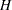be the total number of strata. The total number of replicatesis the smallest multiple of 4 that is greater than. However, if you prefer a larger number of replicates, you can specify the REPS=n method-option. If a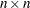Hadamard matrix cannot be constructed, the number of replicates is increased until a Hadamard matrix becomes available.

Each replicate is obtained by deleting one PSU per stratum according to a corresponding Hadamard matrix and adjusting the original weights for the remaining PSUs. The new weights are called replicate weights.

Replicates are constructed by using the firstcolumns of the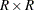Hadamard matrix. Theth (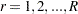) replicate is drawn from the full sample according to theth row of the Hadamard matrix as follows:

• If the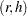th element of the Hadamard matrix is, then the first PSU of stratumis included in theth replicate and the second PSU of stratumis excluded.

• If theth element of the Hadamard matrix is
, then the second PSU of stratumis included in theth replicate and the first PSU of stratumis excluded.

The replicate weights of the remaining PSUs in each half sample are then doubled to their original weights. For more detail about the BRR method, see Wolter (2007) and Lohr (2009).

By default, an appropriate Hadamard matrix is generated automatically to create the replicates. You can display the Hadamard matrix by specifying the VARMETHOD=BRR(PRINTH) method-option. If you provide a Hadamard matrix by specifying the VARMETHOD=BRR(HADAMARD=) method-option, then the replicates are generated according to the provided Hadamard matrix. You can use the VARMETHOD=BRR(OUTWEIGHTS=) method-option to store the replicate weights in a SAS data set.

Let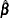be the estimated proportional hazards regression coefficients from the full sample, and let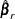be the estimated proportional hazards regression coefficients from theth replicate by using replicate weights. PROC SURVEYPHREG estimates the covariance matrix ofby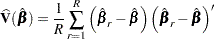withdegrees of freedom, whereis the number of strata.

If one or more components ofcannot be calculated for some replicates, then the variance estimate is computed by using only the replicates for which the proportional hazards regression coefficients can be estimated. Estimability and nonconvergence are the two most common reasons whymight not be available for a replicate sample even ifis defined for the full sample. Letbe the number of replicates whereis available and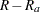be the number of replicates whereis not available. Without loss of generality, assume that the firstreplicates are used; then the BRR variance estimator is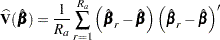with degrees of freedom equal to the minimum ofand, whereis the number of strata. Alternatively, you can use the FAY= method-option to request Fay’s BRR method, as discussed in the following section.

#### Fay’s BRR Method

The traditional BRR method constructs half-sample replicates by deleting one PSU per stratum according to a Hadamard matrix and doubling the original weight of the other PSU. Fay’s BRR method uses the Fay coefficient,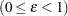, and instead of deleting one PSU per stratum, it multiplies the original weight by the coefficient. The original weight of the remaining PSU in that stratum is multiplied by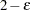. PROC SURVEYPHREG uses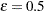as the default value; alternatively, you can specify a value forwith the FAY= method-option. When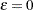, Fay’s method becomes the traditional BRR method. For more details, see Dippo, Fay, and Morganstein (1984); Fay (1984, 1989); and Judkins (1990). Because the traditional BRR method uses only half of the total sample in every replicate, several replicate estimators () might be undefined even when the full sample estimator () is defined. Fay’s BRR method is especially useful for this situation because it uses all the sampled units in every replicate.

Letbe the estimated proportional hazards regression coefficients from the full sample, and letbe the estimated regression coefficients that are obtained from theth replicate by using replicate weights. PROC SURVEYPHREG estimates the covariance matrix ofby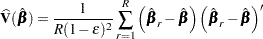withdegrees of freedom, whereis the number of strata.

A Hadamard matrix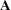of dimensionis a square matrix that has all elements equal to 1 or –1 such that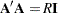, whereis an identity matrix of appropriate order. The dimension of a Hadamard matrix must equal 1, 2, or a multiple of 4.
For example, the following matrix is a Hadamard matrix of dimension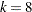:
For BRR replicate construction, the dimension of the Hadamard matrix must be at least, wheredenotes the number of first-stage strata in your design. If a Hadamard matrix of a given dimension exists, it is not necessarily unique. Therefore, if you want to use a specific Hadamard matrix, you must provide the matrix as a SAS data set in the HADAMARD= method-option. You must ensure that the matrix that you provide is actually a Hadamard matrix; PROC SURVEYPHREG does not check the validity of your Hadamard matrix.